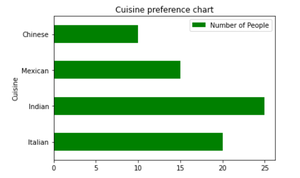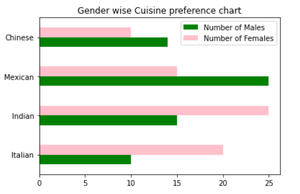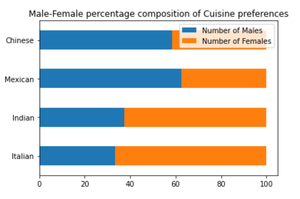# Creating Horizontal Bar Charts using Pandas

• Last Updated : 02 Dec, 2020

Prerequisites: Pandas

A bar chart represents categorical data with corresponding data values as rectangular bars. Usually, the x-axis represents categorical values and the y-axis represents the data values or frequencies. This is called a vertical bar chart and the inverse is called a horizontal bar chart. In some cases, a horizontal bar chart provides better readability.

Python has various visualization libraries such as Matplotlib and Seaborn. The Pandas library, having a close integration with Matplotlib, allows creation of plots directly though DataFrame and Series object. This article explores the methods to create horizontal bar charts using Pandas.

Using the plot instance of the Pandas DataFrame, various kinds of graphs can be created including Bar charts. There are two types of bar charts that represent complex categories:

1. Grouped or Compounded Bar Charts – When you have sub-categories of a main category, this graph assigns each variable or sub-category with a separate bar in the corresponding category.
2. Stacked Bar Charts – When you have sub-categories of a main category, this graph stacks the sub-categories on top of each other to produce a single bar.

The bar() and barh() methods of Pandas draw vertical and horizontal bar charts respectively. Essentially, DataFrame.plot(kind=”bar”) is equivalent to DataFrame.plot.bar(). Below are some examples to create different types of bar charts using the above mentioned functions.

## Simple horizontal bar chart

Syntax:

`DataFrame.plot.barh()`

The barh() methods accept x and y parameters where x takes the categorical values (by default, it takes the index of the DataFrame) and y takes all the numeric columns. The keyword arguments (like title or figure size) supported by DataFrame.plot() can be passed to the barh() method in order to customize the bar chart. Given is the implementation depicting a horizontal bar chart representing the number of people that preferred particular categories of cuisine.

Example:

## Python3

 `# Import required libraries ` `import` `pandas as pd ` ` `  `# Create a sample dataframe ` `df ``=` `pd.DataFrame({``'Cuisine'``: [``'Italian'``, ``'Indian'``, ``'Mexican'``, ``'Chinese'``], ` `                   ``'Number of People'``: [``20``, ``25``, ``15``, ``10``]}) ` ` `  `# Plot a bar chart ` `df.plot.barh(x``=``'Cuisine'``, y``=``'Number of People'``, ` `             ``title``=``'Cuisine Preference'``, color``=``'green'``) `

Output:## Compounded horizontal bar chart

From the above example, if people are divided into sub-groups of males and females then we can represent this data with a compounded horizontal bar chart. This example shows a horizontal bar chart representing the number of males and females that preferred particular categories of cuisine using two methods.

Example 2

Bar chart using barh() method

## Python3

 `# Import required libraries ` `import` `pandas as pd ` ` `  `# Create a sample dataframe ` `df ``=` `pd.DataFrame({``'Number of Males'``: [``10``, ``15``, ``25``, ``14``], ` `                   ``'Number of Females'``: [``20``, ``25``, ``15``, ``10``]}, ` `                  ``index``=``[``'Italian'``, ``'Indian'``, ``'Mexican'``, ``'Chinese'``]) ` ` `  `# Plot grouped horizontal bar chart ` `df.plot.barh(title``=``"Gender wise Cuisine preference chart"``, ` `             ``color``=``{``"green"``, ``"pink"``}) `

Output:Example 3:

Bar chart using plot() method

## Python3

 `# Import required libraries ` `import` `pandas as pd ` ` `  `# Create a sample dataframe ` `df ``=` `pd.DataFrame({``'Number of Males'``: [``10``, ``15``, ``25``, ``14``], ` `                   ``'Number of Females'``: [``20``, ``25``, ``15``, ``10``]}, ` `                  ``index``=``[``'Italian'``, ``'Indian'``, ``'Mexican'``, ``'Chinese'``]) ` ` `  `# Plot stacked horizontal bar chart ` `df.plot(kind``=``"barh"``, title``=``"Gender wise Cuisine preference chart"``, ` `        ``color``=``{``"green"``, ``"pink"``}) `

Output:## Stacked horizontal bar chart

Stacked bar charts are useful in representing the composition or contribution of different sub-groups. The example below shows a horizontal bar chart representing the percentage of males and females that preferred particular categories of cuisine. A stacked horizontal bar chart places the values at each observation in the dataframe side by side in a single bar. However, it stacks the numeric values rather than the percentage of a whole. So, we first convert the data values into the percentage of a whole then use the barh() function with the stacked parameter set to True to create a filled stacked horizontal bar chart.

Example 4

## Python3

 `# Import required libraries ` `import` `pandas as pd ` ` `  `# Create a sample dataframe ` `df ``=` `pd.DataFrame({``'Number of Males'``: [``10``, ``15``, ``25``, ``14``], ` `                   ``'Number of Females'``: [``20``, ``25``, ``15``, ``10``]}, ` `                  ``index``=``[``'Italian'``, ``'Indian'``, ``'Mexican'``, ``'Chinese'``]) ` ` `  `# Convert numeric values to percentage of whole ` `percent_df ``=` `df.``apply``(``lambda` `x: (x ``*` `100``) ``/` `sum``(x), axis``=``1``) ` ` `  `# Plot stacked horizontal bar chart ` `percent_df.plot.barh(stacked``=``True``, ` `                     ``title``=``"Male-Female percentage composition of Cuisine preferences"``) `

Output:My Personal Notes arrow_drop_up
Recommended Articles
Page :